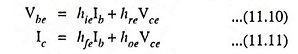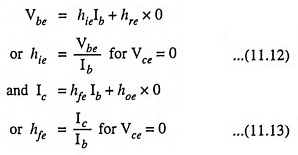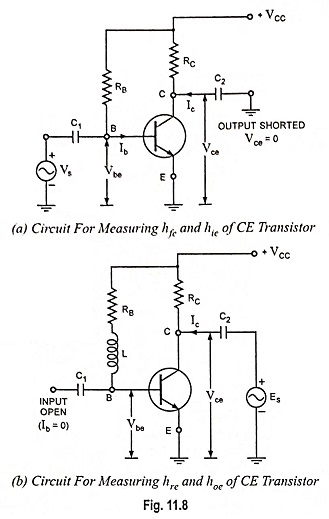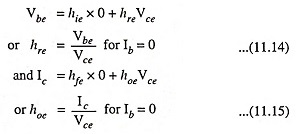## Experimental Determination of Hybrid Parameters:

Determination of hybrid parameters of a general linear circuit has already been discussed. For determination of hybrid parameters for a CE transistor, consider the circuits given in Fig. 11.8. The rms values will be considered in discussion. Volt-ampere equations for a CE transistor are as given below :For measurement of hie and hfe parameters, output is ac short circuited [Fig. 11.8 (a)] by making capacitance C2 deliberately large. The result is that the changing component of collector current flows through C2 instead of RC and ac voltage developed across C2 is zero i.e., Vce = 0. Here it should be clarified that setting Vce = 0 does not mean that the dc collector-emitter voltage, VCE is zero. It simply means that ac output is short circuited.

Substituting Vce = 0 in Eqs. (11.10) and (11.11), we haveThus from Eqs. (11.12) and (11.13) h parameters hie and hfe can be determined. It is to be noted here that Ib and Ic are ac rms base and collector currents respectively. Also Vbe is the ac rms value of base-emitter voltage.

For measuring hybrid parameters hoe and hre the input is ac open circuited, a signal generator is applied across the output and a large inductor L is connected in series with RB, as illustrated in Fig. 11.8 (b). The inductor L, having dc resistance very small, does not disturb the operating point but does not allow flow of ac current through RB. Furthermore, a voltmeter of high input impedance is used to measure base-emitter voltage Vbe and hence there are no paths connected to the base with any appreciable ac current. Thus base is effectively ac open circuited i.e., Ib = 0.Substituting Ib = 0 in Eqs. (11.10) and (11.11), we haveThus by measurement of Vbe, Vce and lc, the hybrid parameters hre and hoe can be determined.

Scroll to Top